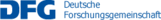## C03 – Nonlinear dispersive equations

Nonlinear waves in diverse areas such as nonlinear optics, large quantum systems and water waves are described asymptotically by dispersive equations, with the cubic nonlinear Schrödinger equation

$i\partial_tu+u_{xx}=\pm|u|^2u$

being perhaps the most prominent among them. This equation, as well as a host of similar dispersive equations, can be solved by the inverse scattering method, which is based on a nonlinear variant of the Fourier transform. The relevance of such integrable equations hinges on a certain robustness: Despite the nonlinearity of the equation, large and highly complex solutions are expected to consist of building blocks described by asymptotic dispersive equations. Of particular interest are properties of solutions to dispersive equations with noise. The goals of this project are threefold.

1. We study solutions to dispersive equations with a focus on harmonic analysis, in particular the robustness of the flow of energy, (scaling) critical regimes and initial data and nonlinear estimates in critical spaces.
2. We study two dimensional water waves with a finite bottom, which seem to be well described by a system of two Korteweg-de Vries equations.
3. We consider stochastic nonlinear wave equations and their large time behavior.

The configurations under study contain a large number of wave packets with controlled self interactions, and we study the interaction with other structures,  either with noise, or other wave packets.

Name Institute Location Phone
Koch, HerbertMIEn60/2.0113787koch@math.uni-bonn.de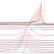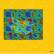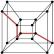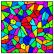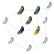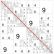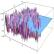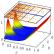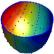## Contact

Coordinator: Prof. Dr. Stefan Müller